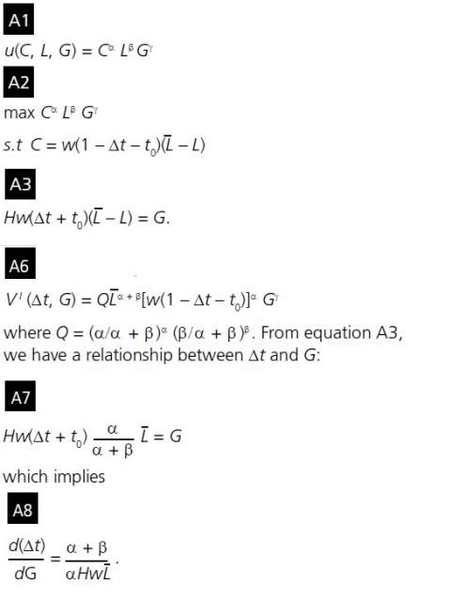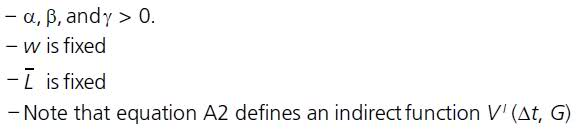# Simple algebra-calculus question

1. Homework Statement , and relevant equations

How is A6 arrived at and *how* did they get the "Q" in A6?## Homework Equations

See above

3. The Attempt at a Solution

Objective: Rid A1 of L. They simply substituted "C" in A2 into A1. But how did they get rid of the L? I don't quite get the algebraic manipulation...please help